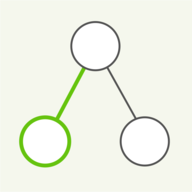17 Apr 2019# Algorithms: Graph search

This page is about algorithms for searching through graphs. Above you see the technique ‘breadth first search’ (BFS) in action. The algorithm is searching for a path through the graph from the solid green node on the left to the solid red node on the right side. Nodes are colored dark green if they have been explored by the search function, together with the edges that have been used to reach them. Note that not all edges are taken, as most nodes can be found from multiple paths. If a path exists from start to finish node, BFS will find it. With this technique, the search is working ‘blind’ i.e. it cannot sense how close it is to the target node. There are techniques that use a so-called heuristic to find the target node, which we will see in a later installment.

The graphs shown here are undirected, planar and have no double edges. Undirected means that the edges can be traversed from both sides, while planar means that you can display the graph on a plane without crossing the edges. No double edges means that two nodes don't have more than one edge between them.## Breadth first search using a queue

Here is a close-up of a smaller graph, to show you what is going on in more detail. The nodes are now numbered to identify them. A queue is shown below the graph, which is full of nodes to be explored. In BFS every new node that is found, is placed at the end of this queue (at the right end). The search moves forward by taking fresh nodes off of the front of this queue (at the left end). If BFS terminates with an empty queue, the start and finish nodes are not in the same connected component of the graph and BFS will have visited all nodes in its own connected component. More about connected components further down this page.## Similarly: Depth first search

The next way to search through a graph, shown above, is to go into depth first, so-called ‘depth first search’ (DFS). If the search reaches a dead end, it picks up further up the path it came down, to find new nodes. DFS discovers all nodes in a connected component, just like BFS, but orders them differently.## Depth first search using a stack

Instead of a queue, which is a first-in first-out data structure, DFS uses a stack, which is a last-in first-out data structure, to store the nodes which should be visited next. The stack is shown below the graph here and grows to the right side.## Application: Connected components

One application of graph search is to identify the various connected components of a graph. If there is no path between two nodes, they are in separate components, i.e. there is a gap. To identify the components we can use either BFS or DFS, but here we are using the latter. Once there are no more nodes to be found by search, a complete component is identified. Simply choose a fresh, unexplored node of the graph and start over to find another component, until all nodes are accounted for. We have implemented this above, with different colors assigned to different components.

Searching graph with BFSTarget node foundTarget node not reachable## Searching directed graphs

The graphs we have considered so far were ‘undirected’, so the edges didn't have a direction and could be traversed from either end. Many practical graphs though are directed, like the example graph for this section. So the edges can only be traversed in direction of the arrows shown. Again, we are doing a BFS search from the solid green node to the red node. Note that this time, the search often stalls with no further nodes that can be explored. This is due to the fact that the directed graph has fewer possible paths to explore.

Showing cycles in a directed graph

## Cycles in directed graphs

Directed graphs, in general, can have cycles in them. This means that you can find a closed loop, following the right direction of each edge, back to the starting point. The animation above shows the cycles that have been found in the graph. It is a desirable thing to have a graph free of cycles, which is the topic of the last panels below.

A directed acyclic graph (DAG)

## Directed, acyclic graphs

Now that we've seen graphs with cycles, here is an example of a graph with no cycles, called a directed, acyclic graph, or simply DAG. We have generated one by making sure all edges have their direction pointing to the right, which prevents cycles from occuring. A DAG has at least one source, which is a node with only outgoing edges. The sources are shown in green above. A sink is a node with only incoming edges, and these are colored in red. DAGs have certain other nice properties, for example they admit a so-called topological sort or topological ordering, which is the topic of the next section.## Application: Topological sort

A topological sort is a linear arrangement of the nodes of a DAG, such that all edges in the graph point to the right, in one direction. In other words, there are no backward-directed edges, and hence no cycles. Finding a topological sort is fairly simple: execute a (recursive) DFS, and every time all descendant nodes of a node have been fully processed, assign the next ordering number to that node, starting from the last node and counting backwards. This is what the numbers in the nodes stand for. Start the DFS from every source node, until all nodes have been processed. Note that there can be more than one topological ordering for a DAG.

Stay tuned for more pages on graph topics, and have a look at the main page for other topics.